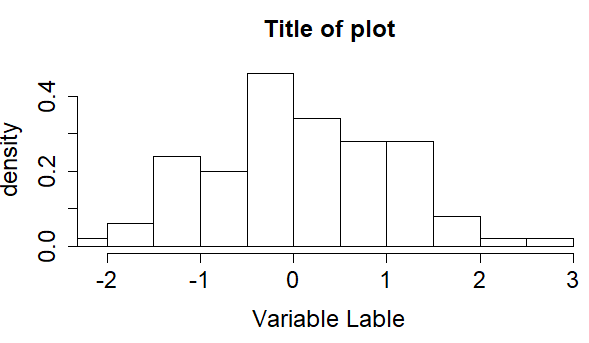2 views

I am confused. What is the right way to increase the font size of the text in the title, labels and other places of a plot?

For example

x <- rnorm(100)

hist(x, xlim=range(x), xlab= "Variable Label",

ylab="density", main="Title of plot", prob=TRUE, ps=30)

The ps argument does not change the font size (but it says in R Help for ?par that it is for "the point size of text (but not symbols)".

Also is it possible to separate changing the font size from the plotting function such as hist?

by

To magnify the font size in your plots, use the cex argument in the following ways:

cex

A numerical value giving the amount by which plotting text and symbols should be magnified relative to the default. This starts at 1 when a device is opened and is reset when the layout is changed, e.g. by setting mfrow.

cex.axis

The magnification to be used for axis annotation relative to the current setting of cex.

cex.lab

The magnification to be used for x and y labels relative to the current setting of cex.

cex.main

The magnification to be used for main titles relative to the current setting of cex.

cex.sub

The magnification to be used for subtitles relative to the current setting of cex

To magnify to 150 percent.

x <- rnorm(100) hist(x, xlim=range(x), xlab= "Variable Lable", ylab="density", main="Title of plot", prob=TRUE, cex.lab=1.5, cex.axis=1.5, cex.main=1.5, cex.sub=1.5)

Output: